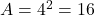## A cube has a volume of 64 cubic feet. What is the AREA of one of the sides?

Question

A cube has a volume of 64 cubic feet. What is the AREA of one of the
sides?

in progress 0
1 month 2021-08-13T20:50:33+00:00 1 Answers 3 views 0

16 square feet

Step-by-step explanation:

First, let’s find how long each side of the cube is.

The volume of a cube is found by this formula, where s is a side of the cube: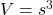We can take the cube root of both sides: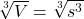Just flipping it: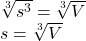We know our volume is 64 cubic feet. Let’s put that into our equation as V: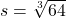The cubic root of 64 is 4, since 4 * 4 * 4 = 64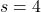Thus, each side of our cube is 4 feet.

Now, the area of a side of our cube is found using this formula: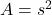Since we know s, we can easily find the area A by squaring it: